# Quadratic Equations: Find Factors QuicklyQuadratic equations – These are the equations which look like

ax2 + bx + c = 0
These equations are asked in the competitive examinations in a set of 5 questions. Enough with the theory, the question in exams look like -
In each of the following questions, two equations are given. Solve these equations and give the answer:
A) If x >= y, i.e. x is greater than or equal to y
B) If x > y, i.e. x is greater than y
C) If x <= y, i.e. x is less than or equal to y
D) If x < y, i.e. x less than y
E) If x = y, or no relation can be established between x and y

### Ques 1

I. x2 + 5x + 6 = 0
II. y2 +7 y + 12 = 0

Here we will discuss a method to solve these equations with minimal writing and as reduced time as possible. Keep in mind; we are doing exactly what we used to do before, just in a less time and less writing.
Older way

x2 + 5x + 6 = 0
We used to solve it as
x2 + 3x + 2x + 6 = 0
x(x + 3) + 2(x + 3) = 0
(x + 3) (x + 2) = 0
Then, we get the two roots which are x = -3, -2

Let’s try all of this but another way.
Consider the same equation:

x2 + 5x + 6 = 0
When we compare this equation to
ax2 + bx + c = 0
we get, a = 1, b = 5, c = 6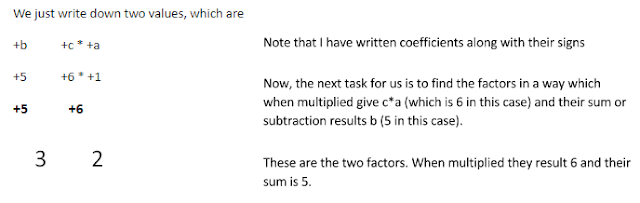#### A way to find the factors

• Start dividing the number formed using “c*a” starting from first prime number i.e. 2. The result gives one factor and the other factor is the number you divided with.
• Add them and then subtract them to see if they give “b”.
• If not divide it with next number on number line i.e. 3 and so on till you get the final factors.
• Don’t worry this method seems lengthy but with a little bit of practice it will get faster.

We have our factors 3 and 2 and now is the time to decide the sign. For that, riddle me this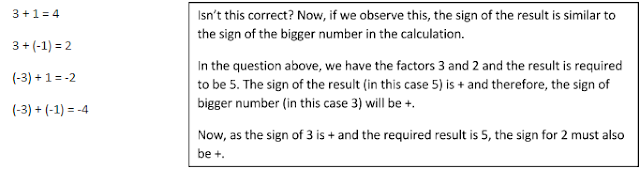Therefore, the factors become +3, +2

#### Remember two things before marking them as final answers:

• Change the sign of the factors i.e. – if they are + and + if they are -.
• Divide the two factors by “a”.
In the above question, the value of “a” is 1. Therefore, the factors become
3/1 = 3 2/1 = 2

And for the last touch, we change the sign. After that, we get the final factors.

Final Factors: -3, -2
Let’s take one more example to make process more familiar to you.
x2 – 3x – 10For the last touch, we change the signs and divide them with “a”, which in this case is 1.
Final Factors: -2, 5

Hopefully, the method was crystal clear to you and comparatively easy then the traditional method. Let’s now take a question similar to the one asked in the examination.

In each of the following questions, two equations are given. Solve these equations and give the answer:
A) If x >= y, i.e. x is greater than or equal to y
B) If x > y, i.e. x is greater than y
C) If x <= y, i.e. x is less than or equal to y
D) If x < y, i.e. x less than y
E) If x = y, or no relation can be established between x and y

## Examples

#### Ques 1

I. x2 + 6x + 9 = 0
II. 2y2 +3y - 5 = 0
x2 + 6x + 9 = 0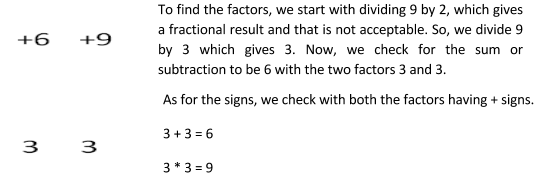For the two unforgettable things to do i.e. division by “a” (1 in this case) and change of signs.
Values of x -3, -3

For equation no. 2
2y2 +3y - 5 = 0
Just a last tiny step, division by “a” (2 in this case) and change of sign.

5/2 = 2.5, 2/2 =1

Values of y: -2.5, 1

We compare every value of x to every value of y and note the results down.
 Value of x Value of y Conclusion -3 (1st value) -2.5 x < y -3 (1st value) 1 x < y -3 (2nd value) -2.5 x < y -3 (2nd value) 1 x < y

As in all the cases, x < y, we can say with certainty that x < y (final answer).

## Practice Questions

In each of the following questions two equations are given. Solve these equations and give answer:
A) If x >= y, i.e. x is greater than or equal to y
B) If x > y, i.e. x is greater than y
C) If x <= y, i.e. x is less than or equal to y
D) If x < y, i.e. x less than y
E) If x = y, or no relation can be established between x and y

Q 1:
I. x2 + 5x + 6 = 0
II. y2 +7 y + 12 = 0Now, we compare
 Value of x Value of y Conclusion -3 -4 x > y -3 -3 x = y -2 -4 x > y -2 -3 x > y
We can say with certainty that x >= y (final answer).

Q 2:
I. x2 + 4x + 4 = 0
II. y2 – 8y + 16 = 0Now, we compare
 Value of x Value of y Conclusion -2 (1st factor) 4 (1st factor) x < y -2 (1st factor) 4 (2nd factor) x < y -2 (2nd factor) 4 (1st factor) x < y -2 (2nd factor) 4 (2nd factor) x < y
We can say with certainty that x < y (final answer).

Q 3:
I. x2 – 19x + 84 = 0
II. y2 – 25y + 156 = 0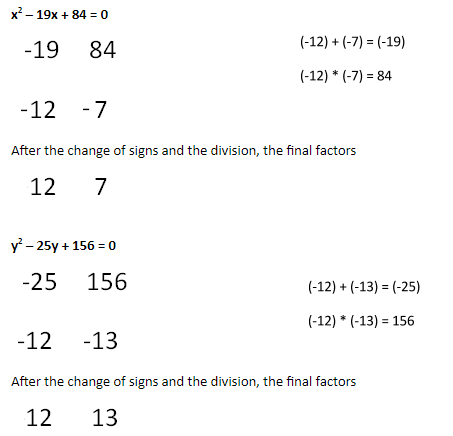Now, we compare
 Value of x Value of y Conclusion 12 12 x = y 7 12 x < y 12 13 x < y 7 13 x < y
We can say with certainty that x <= y (final answer).

Q 4:
I.4x2 – 8x + 3 = 0
II.2y2 – 7y + 6 = 0Now, we compare
 Value of x Value of y Conclusion 1.5 2 x < y 0.5 2 x < y 1.5 1.5 x = y 0.5 1.5 x < y
We can say with certainty that x <= y (final answer).

Q 5:
I. x2 + x – 6 = 0
II.2y2 – 13y + 21 = 0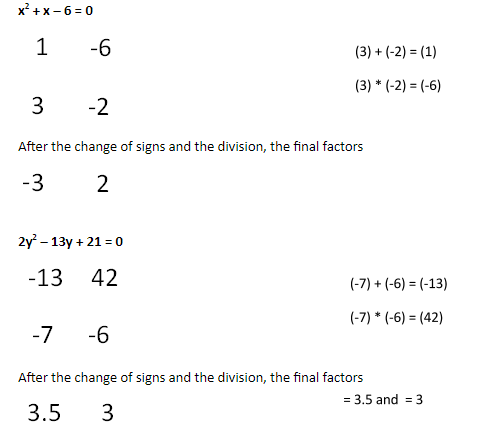Now, we compare
 Value of x Value of y Conclusion -3 3.5 x < y 2 3.5 x < y -3 3 x < y 2 3 x < y
We can say with certainty that x < y (final answer).

## Exercise For Practice

Q 1: I. x2 – x – 6 = 0
II. 2y2 + 13y + 21 = 0

Q 2: .I. x2 + 5x + 6 = 0
II. y2 + 3y + 2 = 0

Q 3: I. x2 + x = 56
II. y2 – 17y + 72= 0

Q 4: I. 3x2 + 17x + 10 = 0
II. 10y2 + 9y + 2 = 0

Q 5: I. 12x2 + 11x + 12 = 10x2 + 22x
II. 13y2 – 18y + 3 = 9y2 – 10y

Q 6: I.3x2 – 19x + 28 = 0
II.5y2 – 18y + 16 = 0

Q 7: I. 6x2 + 5x + 1 = 0
II. 15y2 + 8y + 1 = 0

Q 8: I. x2 + 5x + 6 = 0
II. 4y2 + 24y + 35 = 0

Q 9: I. 2x2 + 5x + 3 = 0
II. y2 + 9y + 14 = 0

Q 10: I. 88x2 – 19x + 1 = 0
II. 132y2 – 23y + 1 = 0